# Post-processing quantities

## Introduction

The post-processing quantities are of two types:

• local quantities, analyzed in all points of the study domain,
• global quantities*, resulting from an integration, analyzed over the entire study domain or on a part of this domain.
Note: * In the presence of symmetries and/or periodicities these quantities are computed for the part of the device represented in the finite elements domain.

## Usual quantities

Usual local quantities available are presented on the table below.

Usual quantities

Flux

formula

Flux Unit Explanation Application
2D plane 2D axi 3D
Temperature Temp(Tkelvin) K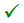Temperature (gradient) mGradT K/mHeat capacity (volume): ρCP RhoCp J/(m3 .K)Thermal conductivity: k Kth W/(m.K)Thermal flux (surface density):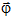dFluxTh W/m2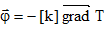or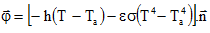*Usual local quantities available in advanced use are presented on the table below.

Quantities

Flux formula Flux Unit Explanation Application
2D plane 2D axi 3D
Temperature (Kelvin): TKelvin TKelvin KTemperature (Celsius): TCelsius TCelsius °C TCelsius = TKelvin -273.15Heat (volume density): q dHeatV W/m3Heat (surface density): q dHeatS W/m2Heat (line density): q dHeatL W/m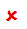Heat transfer (convection coefficient): h Hconv W/(m2.K)Hconv2s (on both sides, for regions with double heat exchange)Heat transfer (radiation coefficient): ε Hrad W/(m2 .K4)Hrad2s (on both sides, for regions with double heat exchange)Ambiante temperature

(for convectif exchange)

Tamb KTamb2s (on both sides, for regions with double heat exchange)Heat transfer (surface density):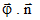dExchangeS W/m2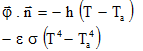*Note: * If the local radiation is applied, the second term is to replace by the thermal flux expression given in the part 1.1.6 of this document.

## Global quantities

Global quantities are not directly available. They can be calculated by integration (See § Explanation of results)